# How to Calculate SMAPE in Excel?

• Last Updated : 28 Jul, 2021

In statistics, we often use Forecasting Accuracy which denotes the closeness of a quantity to the actual value of that particular quantity. The actual value is also known as the true value. It basically denotes the degree of closeness or a verification process that is highly used by business professionals to keep track records of their sales and exchanges to maintain the demand and supply mapping every year. There are various methods to calculate Forecasting Accuracy.

So, one of the most common methods used to calculate the Forecasting Accuracy is MAPE which is abbreviated as Mean Absolute Percentage Error. It is an effective and more convenient method because it becomes easier to interpret the accuracy just by seeing the MAPE value.

Attention reader! Don’t stop learning now. If you are an Excel beginner (or an intermediate) and want to learn Excel, Geeksforgeeks brings the perfect course for you to start, Diving Into Excel

Symmetric Mean Absolute Percentage Error abbreviated as SMAPE is also used to measure accuracy on the basis of relative errors. It is basically a prediction accuracy technique an alternative to MAPE. It is generally represented in terms of percentage (%).

In this article we are going to discuss how to calculate SMAPE in Excel using a suitable example.

The formula to calculate SMAPE in Excel is :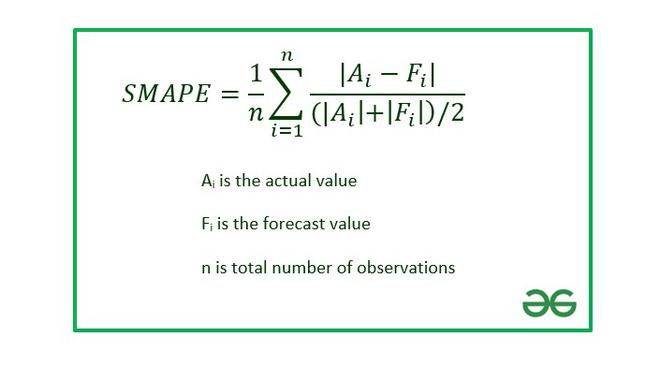The above formula gives a lower bound value of 0% and upper bound value of 200%.

Note :

1. The above formula becomes invalid when both the Actual value and the Forecast value tend to zero.
2. Due to the presence of upper and lower bounds it provides more symmetric results which is not present in MAPE.
3. Generally, we prefer the percentage error between 0% to 100%. So, the term division by 2 is eliminated and the above formula is modified to calculate SMAPE.

Example: Consider the dataset shown below :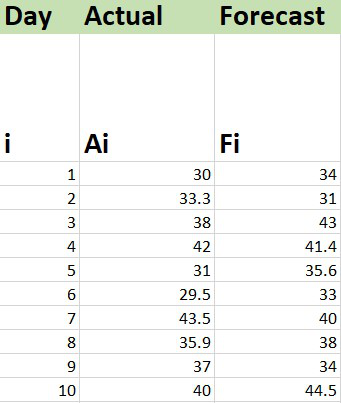## Calculation of SMAPE

The functions needed for formulas in Excel are-

ABS : To calculate the absolute value.

AVERAGE : To calculate the mean.

The steps are :

1. Insert the data set in the Excel sheet.

2. Calculate the subpart of the formula inside the summation which is also known as SMAPE difference.

```=2*ABS(Cell_No_Act-Cell_No_Fore)/(ABS(Cell_No_Act)+ABS(Cell_No_Fore))

where
ABS : Used to calculate the absolute value
Cell_No_Act : Cell number where Actual value is present
Cell_No_Fore : Cell number where Forecast value is present```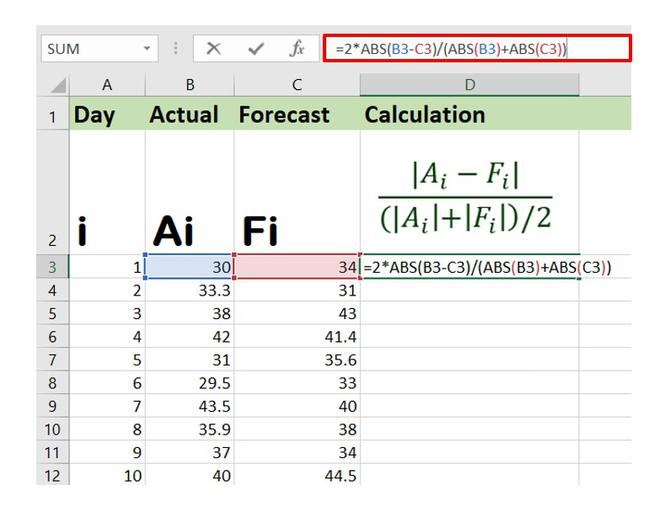The above formula will calculate the SMAPE difference for the first entry in the data set. Similarly, you can drag the AutoFill Options button to get the SMAPE difference for the entire dataset.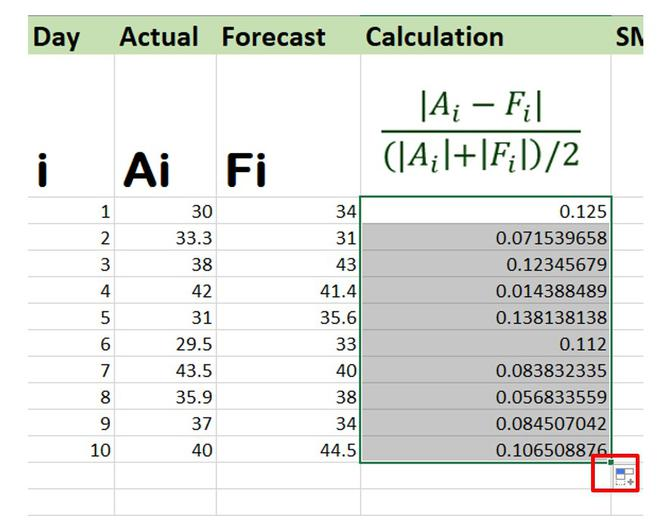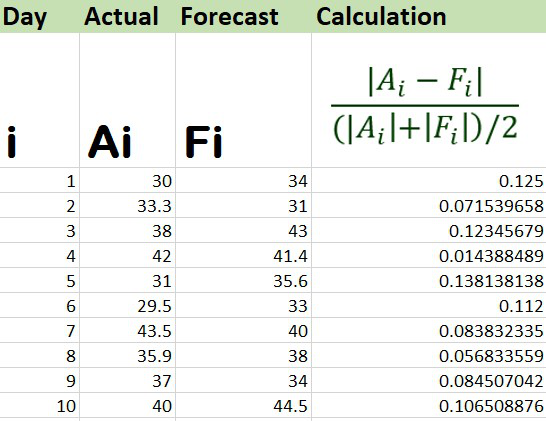SMAPE Difference

3. Now, simply take the mean or the average value of all the data obtained in step 2 using the Excel AVERAGE formula. The syntax is :

`=AVERAGE(Cell_Range)`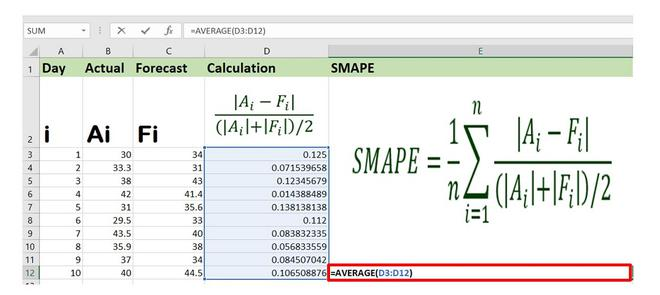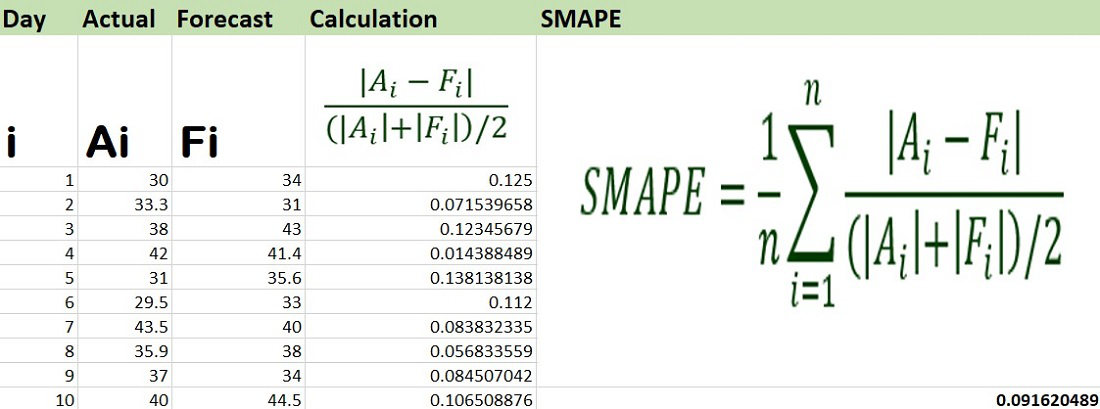Therefore, the value of SMAPE for the given dataset is 0.0916 or 9.16%.

My Personal Notes arrow_drop_up